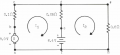# Solving network problems w/ kirchoff's law (mesh current method)

#### meanface

Joined Apr 9, 2015
9
I'm understanding everything until its gets to the part where it states to "Equations (1) AND (2) may be solved simultaneously by multiplying Equation (2) by 3 and adding the result to Equation (1)::"
Why 3, Where are we getting the 3 from ?To write an equation for the first mesh, select a convenient starting point such as point a and go around the mesh clockwise, following the direction of I1. Since the first circuit element encountered is the generator, and since the tracing direction passes through the ernf from the negative to the positive terminal, the first term of our equation should be written -8. A drop of +0.1I1 occurs from points b to c, and another drop of +0.05I1 occurs between points d and e.

We next encounter the positive terminal of the battery which should be accounted for in the equation by the term +6.4. On emerging from the battery we arrive at point f which is the same as point a and our traversal of Mesh 1 appears to be complete. At this point, we could very easily make the mistake of writing the equation for the first mesh as follows:
-8 + 0.1I1 + 0.05I1 + 6.4 = 0

However, an extremely important point has been overlooked! Although current I1 flows downward between points d and e, this is not the only current flowing between these points. Current I2, in the adjacent mesh, also flows between these same points and I2 flows upward from e to d contrary to the direction given I1. Since the drop caused by I2 is in opposition to the drop caused by I1, this serves to reduce the total drop caused by I1 and must be indicated in the equation of the first by a minus sign. The complete equation for Mesh 1 then becomes:
-8 + 0. 1I1 + 0.05I1 - 0.05I2 + 6.4 = 0
Or by collecting terms and transposing
0.15I1 - 0.05I2 = 1.6 (1)

Turning to Mesh 2, start at point f and traverse the loop fdgh, following the direction I2, writing the signs of the emfs according to the signs of the terminals first encountered and considering all drops caused by I2 to be positive. This time, any drops caused by I1 carry a negative sign since they are in opposition to the drops caused by I2

The complete equation for Mesh 2 is then,

-6.4 + 0.05I2 - 0.05I1 + 0.5I2 = 0
collecting terms and transposing
-0.05I1 + 0.55I2 = 6.4 (2)

Equations (1) and (2) may be solved simultaneously by multiplying Equation (2) by 3 and adding the result to Equation (1):
-0.15I1 - 0.05I2 = 1.6 (1)
-0.15I1 + 1.65I2 = 19.2 (2)

1.60I2 = 20.8

I2 = 13 amps

#### Jony130

Joined Feb 17, 2009
5,351
You do not know haw to solve simultaneous equations ??
You simply substitute 13A into equation (1) or (2) and solve for I1

#### meanface

Joined Apr 9, 2015
9
I guess you didnt read the question.

#### Dodgydave

Joined Jun 22, 2012
10,363
where do you get this " multiply by 3" from.

#### meanface

Joined Apr 9, 2015
9
where do you get this " multiply by 3" from.

Lol exactly ! Why 3 and where is the 3 coming from ?

#### WBahn

Joined Mar 31, 2012
26,487
Are you familiar with the concepts associated with solving simultaneous equations? This is generally expected well before you get to using mesh analysis, so you probably have some work to do to come up to speed -- and if you don't it's only going to get worse.

Look at the two equations you have;

Mesh 1: 0.15I1 - 0.05I2 = 1.6
Mesh2: -0.05I1 + 0.55I2 = 6.4

As painful as it is, I will leave aside the issue of the author's failure to properly track their units and just go with what they have.

The goal is to combine these two equations in such a way that one of the variables drops out.

If we multiply the bottom equation by 3 (which is obtained by 0.15/0.05), then the coefficients of the I1 terms are the same except opposite in sign. Thus when we add them the I1 terms do not survive. We could have also multiplied the top equation by 11 (0.55/0.05) and added them together to make the I2 term disappear.

#### meanface

Joined Apr 9, 2015
9
Are you familiar with the concepts associated with solving simultaneous equations? This is generally expected well before you get to using mesh analysis, so you probably have some work to do to come up to speed -- and if you don't it's only going to get worse.

Look at the two equations you have;

Mesh 1: 0.15I1 - 0.05I2 = 1.6
Mesh2: -0.05I1 + 0.55I2 = 6.4

As painful as it is, I will leave aside the issue of the author's failure to properly track their units and just go with what they have.

The goal is to combine these two equations in such a way that one of the variables drops out.

If we multiply the bottom equation by 3 (which is obtained by 0.15/0.05), then the coefficients of the I1 terms are the same except opposite in sign. Thus when we add them the I1 terms do not survive. We could have also multiplied the top equation by 11 (0.55/0.05) and added them together to make the I2 term disappear.

Thank You
I just didnt understand where this "3" came from.